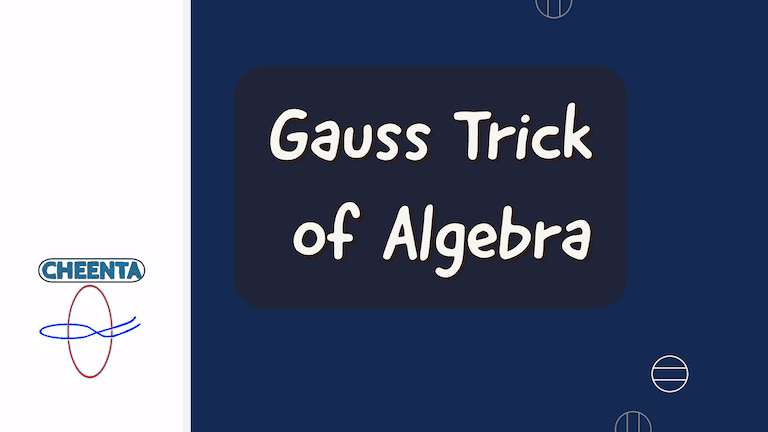How Cheenta works to ensure student success?
Explore the Back-Story

# Gauss Trick in ISI Entrance## Problem - Gauss Trick (ISI Entrance)

Let's learn Gauss Trick for ISI Entrance.

If k is an odd positive integer, prove that for any integer $\mathbf{ n \ge 1 , 1^k + 2^k + \cdots + n^k }$ is divisible by $\mathbf{ \frac {n(n+1)}{2} }$

### Key Concepts

Gauss Trick

Factoring Binomial

## Source

From I.S.I. Entrance and erstwhile Soviet Olympiad.

Test of Mathematics at 10+2 Level by East West Press, Subjective Problem 31

Challenges and Thrills of Pre College Mathematics

## Problem - Gauss Trick (ISI Entrance)

Let's learn Gauss Trick for ISI Entrance.

If k is an odd positive integer, prove that for any integer $\mathbf{ n \ge 1 , 1^k + 2^k + \cdots + n^k }$ is divisible by $\mathbf{ \frac {n(n+1)}{2} }$

### Key Concepts

Gauss Trick

Factoring Binomial

## Source

From I.S.I. Entrance and erstwhile Soviet Olympiad.

Test of Mathematics at 10+2 Level by East West Press, Subjective Problem 31

Challenges and Thrills of Pre College Mathematics

## Subscribe to Cheenta at Youtube

This site uses Akismet to reduce spam. Learn how your comment data is processed.

### Knowledge Partner